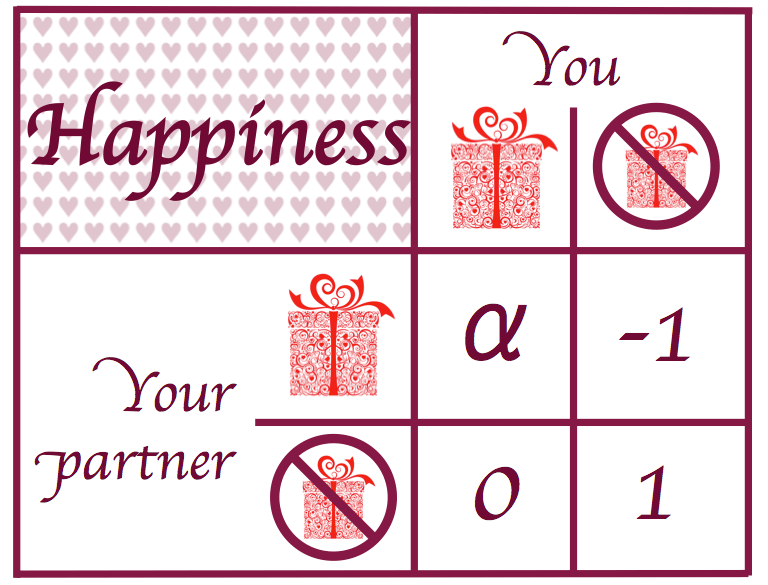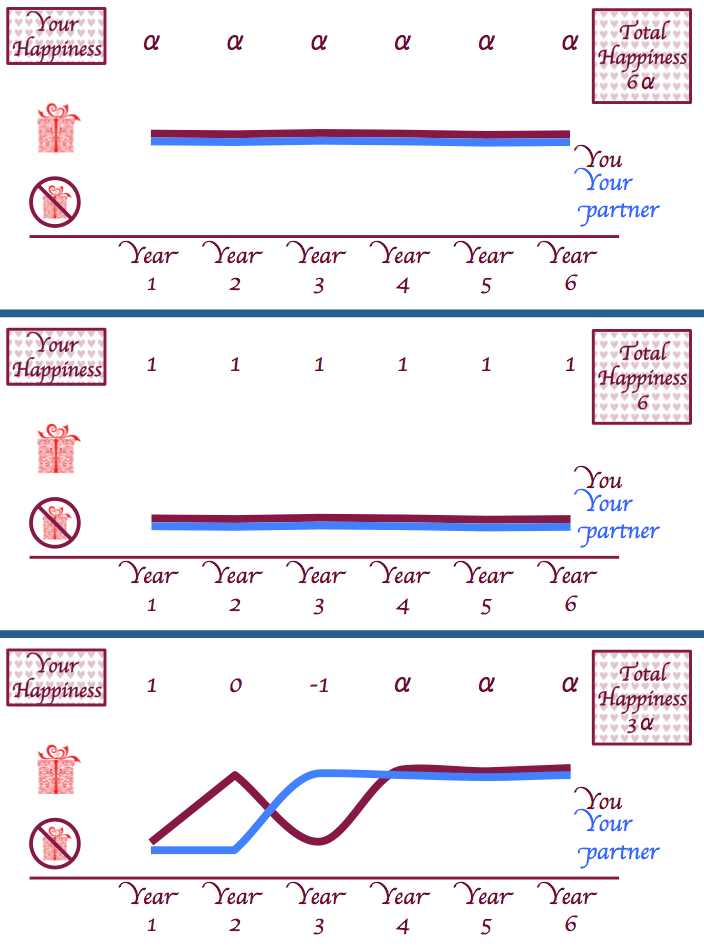# Should you buy a Valentine’s day present?Valentine’s day is just around the corner and you are still not sure whether or not you should buy your beloved one a present.  It’s a tough call. Should you spend money on buying your partner some chocolates and a teddy bear (that no one wants anyway), or will you risk it and bring only a charming smile to your romantic dinner?

A tough one, as we said! Firstly, if you don’t bring a present and you don’t get one either, then nothing will have changed, both of you will probably be just as happy (probably more: it’s Valentine’s) as you were before and you both have the added bonus of having saved some money. Awesome!  Super awesome, in fact! But if you don’t bring a present and your partner does, you will end up feeling miserable the whole night. You’ll find yourself repeating “I should have bought those chocolates” to yourself, when you would really much rather be repeating something else.

On the other hand, if you actually buy a present and you get one in return, you’ll both feel happy (although with less money in your pockets). There is also, of course, the chance that you bring your present and get nothing in return. Sad times for you but, nonetheless, not as miserable as the case where you’re the one who forgot the present.What a mess! You wander around the endless corridors of teddy bears, chocolates and teddy bears made out of chocolate wondering whether you should buy any of those items. “This is all commercialism,” you think to yourself every time you are about to grab one of those Valentine’s cards. “We should celebrate love every day.” But then, when you leave the shop in disgust, you find that everything is covered in red ribbons and baby cupids, reminding you that you still haven’t got a present for your beloved one. The day is nearly there and you still haven’t figured out whether you should buy something or not!

Worry not! Maths is here to help you! Let’s write your happiness, $H$, in terms of a pay-off matrix: the scenarios are bringing a present or not and receiving one or not.The table above summarises the happiness level ($H$) we will experience given the different scenarios. Or at least, based on the reasoning given above (into which much thought and time was invested), it’s what the authors feel is realistic.  Different people might quibble with the relationship of each entry to the other: we don’t care.  Note that we’ve kept the happiness derived from both buying each other presents ($\alpha$) as a variable—because, if we have to be honest, we disagreed on which was the best scenario (and keeping $\alpha$ as unknown leads to an interesting conclusion). So, with this information, the question is: to buy or not to buy?

## Coming up with a strategy

A reasonable strategy is to choose the option that gives you the highest expected level of happiness $H$. Assume, for now, that the probability of your partner buying you a present is $p$. Therefore, if you buy a present your expected level of happiness is

$$E(H) = \alpha p;$$

and if you decide not to buy  then

$$E(H) = 1 – 2p.$$

So we would like to buy the present if our expected level of happiness when we buy the present is greater than when we don’t, giving $p>1/(2+\alpha)$. The strategy is therefore as follows: if $p > 1/(2+\alpha)$, we buy the present and our expected happiness is $\alpha p$; if $p < 1/(2+\alpha)$, we don’t buy the present and our expected happiness is $1 – 2p$.  This is plotted on the graph: quite clearly, neither of us should buy. But, it’s love and love is irrational, so how on earth are we supposed to know what $p$ is?Expected happiness $H$ of buying a present. Don’t buy!

## Finding $p$

Buying a present for our partners is, we decided, an iterative process: what I decide to do one year is likely to be based on what my partner did in the past.  If they tend not to buy me a present, then maybe I’d be tempted not to get them one either—because love is vengeful, right?  We can set up a simple model to represent this.  Again, $p$ represents the probability of my partner buying me a present and $q$ is the probability of me buying my partner a present; except that now we make them discrete variables, so $p_n$ is the probability of my partner buying me a present in year $n$ (similarly for $q_n$).  We also introduce the variables $x_n$ and $y_n$, where $x_n, y_n = 1$ if I/my partner buys me a present in year $n$, and 0 otherwise.

The strategy for deciding whether to buy a present or not remains the same as that described above:

$$x_n = 1 \iff p_{n} > \frac{1}{2 + \alpha} \quad \text{and} \quad y_n = 1 \iff q_n > \frac{1}{2 + \alpha}.$$

How do we compute the probabilities $p_n$ and $q_n$?  In the natural way: assume that our memory is of $m$ years, ie we both remember what the other did for the past $m$ years.  The probability is then

$$p_n = \frac{\sum_{i=n-m+1}^{n-1} y_i}{m}$$

and $q_n$ has a similar formula, with $y_i$ replaced by $x_i$.  In the first $m$ years, the probability is derived from the existing data (but this isn’t overly important for our article).

What happens?  The case where we both buy each other a present is easy: both $p_n$ and $q_n$ are equal to one for all time, which is obviously greater than $1/(2+\alpha)$ and so we will always buy each other a present.  If, in the first year, neither of us buys the other a present (the optimal scenario if $\alpha < 1$), then, using a similar argument, neither of us ever will…

But what if, for some bizarre reason—something to do with love, say—you decide one year to buy your partner a present (or vice versa: the situation is symmetric)?  Then the probability of you buying your partner a present the next year increases from zero to $1/m$.  My partner then has a decision to make: s/he will buy me a present if

$$\frac{1}{m} > \frac{1}{2+\alpha} \iff m < 2 + \alpha.$$

If this condition is satisfied, they buy me a present; I don’t (because their probability remains zero) and we’re doomed.  The year after, assuming $m \geq 2$ (if $m = 1$, everything is trivial), my probability remains $1/m$, my partner’s updates to $1/m$ too, we both buy each other presents and continue to do so until the end of time (which is obviously more likely to happen than us breaking up).We both buy; neither of us buys; the inevitable occurs… If it’s your first date you might as well just bite the bullet.

So it all hinges on the values of $m$ and $\alpha$.  The latter is always positive (it’d be a slightly perverse couple that feels sad at the fact that they’ve bought each other presents); and, given that I can’t remember what I had for dinner last night, $m$ must be equal to 2, so here’s the f*cking wrapping paper…At least with this wrapping paper, you can have a little fun and say that it was randomly generated… pretty much like your gift selection at the shop.

[Pictures: 1 – adapted from Flickr.com – Burning for Love by John Chandler, CC-BY 2.0; 2 – from Flickr.com – a box of chocolates by Casey Bisson CC-BY 2.0; other pictures by Chalkdust]Rudolf Kohulák is a PhD student at UCL working on the modelling of freeze-drying processes.Pietro is interested in history and sport. He also happens to be doing a PhD in fluid dynamics at UCL. If he can combine any two of the three it makes him a happy man.Rafael Prieto Curiel is doing a PhD in mathematics and crime. He is interested in mathematical modelling of any social issues, such as road accidents, migration, crime, fear and gossip.Alex Doak is a PhD student at UCL researching fluid mechanics.
alexander.doak.11@ucl.ac.uk